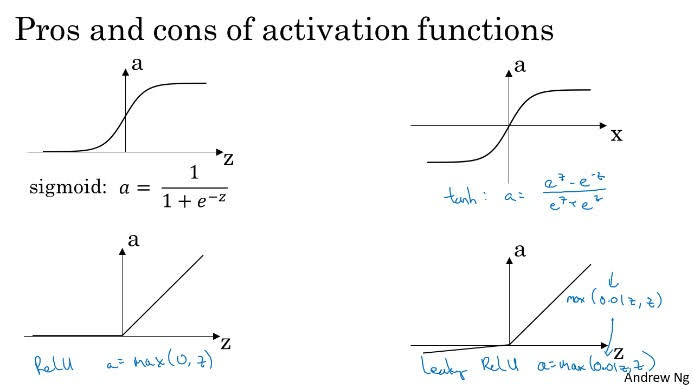Categories:

Updated:

# Why Use Non-Linear Activation Function?

The reason we used hidden layers was to represent complex, non-linear model. Non-linear activation function adds this complexity to the model.

If we don’t use activation or use linear activation function, our final model can be represented with a single linear regression or logistic regression.

# Pros and Cons of Activation Function• Tanh function is always faster than sigmoid function.
• With sigmoid and tanh, when absolute value of z becomes big, slope of function converges to 0, so gradient descent becomes slower.
• Most times, we use ReLU which is faster than tanh
• Try using Leaky ReLU
• Sigmoid is commonly used as an activation function of output layer in binary classification

Categories: